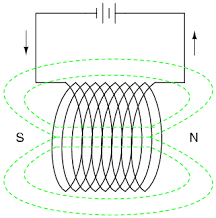# What is Field in Physics, Types Of Field in Electrical System

## What is Field:

Field is a physical quantity, which is represented by a number There are many types are field in physics. Lets see..

## Electric Field:

Electric Field: When an electric charge is surrounded by another electric charge in the same region means, it experiences a force.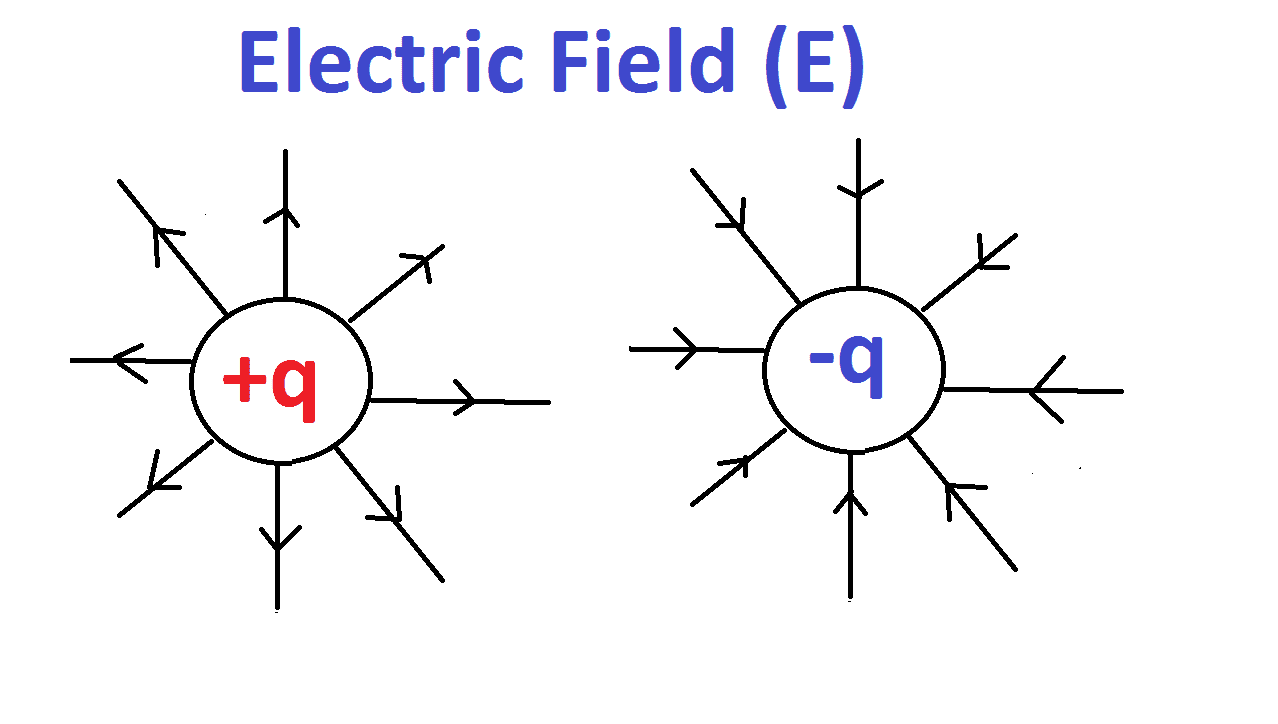We know that electric charges experience forces in the presence of other charges. An electric charge induces an electric field in the space surrounding it which in turn exerts forces on other charges present.

The force F is acting on a charge q in an electric field E is given by..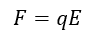The magnitude of the electric field due to a point charge q at a distance r away from the point charge is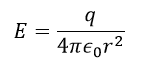This electric field acts radially outwards for a positive charge and radially inwards for a negative charge.

## Gravitational Fields:

Example: Objects fall to the ground because they are affected by the force of earth’s gravitational field.

Newton’s Law of Gravitation states that the gravitational force F between two point masses M and m a distance r apart in a vacuum is attractive, acts along the line joining their centers, and is proportional to the masses and inversely proportional to the square of their separations.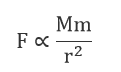F is the gravitational force, M and m = Mass

In the SI system, the constant of proportionality is G, the gravitational constant, which has a value of 6.67×10−11Nm2kg−2, and so we may write this as,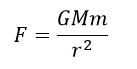The gravitational field is the gravitational force per unit mass that would be exerted on a small (so it doesn’t measurably affect the gravitational field) test mass at that point. It is a vector field, and points in the direction of the force that a small test mass would feel at that point. For a point particle of mass M, the magnitude of the resultant gravitational field strength g, at distance r from M, is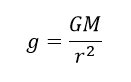The gravitational force acting on a mass m, which is also sometimes described as its weight in the gravitational field g, is given by:## Magnetic Field:

Example: A paper clip, placed in the magnetic field surrounding a magnet, is pulled toward the magnet, and two like magnetic poles repel each other when one is placed in the other’s magnetic field.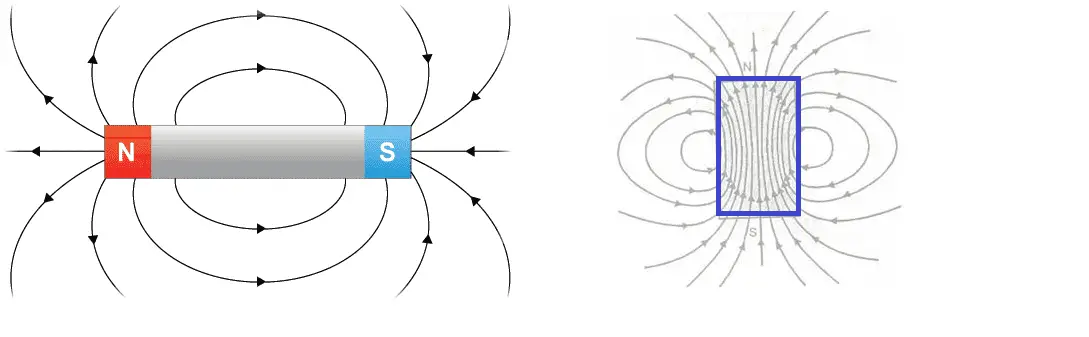A magnetic field can be produced by either a current flow (movement of charge) or a magnetized material. The pole of a magnet or a moving charged particle in a magnetic field will feel a magnetic force.

## Electromagnetic Field:

Electromagnetic field, a property of space caused by the motion of an electric charge. A static charge will produce only an electric field in the surrounding space (That is voltage). Also, If the same charge is moving around the conductor (that is current), it produces a magnetic field. An electric field can be produced also by a changing magnetic field. The mutual interaction of electric and magnetic fields produces an electromagnetic field, which is considered as having its own existence in space apart from the charges or currents (a stream of moving charges) with which it may be related.

Best example: Inductor. An inductor is connected across the voltage source. It stores energy and releases the same.